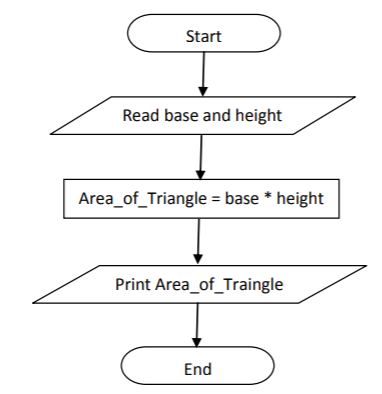# O Level Exam

Question. What is algorithm? Write the characteristics of an algorithm. Give an example for algorithm.

Definition of Algorithm

Algorithm is a step by step solution to a given problem. Sequence of steps written in order to carry out some particular task. Each step of an algorithm is written in english.

Various characteristics of an algorithm are

• The algorithm must have definite start and end.
• An algorithm may accept zero or more inputs
• An algorithm must produce at least one output
• The steps of an algorithm must be simple, easy to understand and unambiguous.
• Each step must be precise and accurate.
• The algorithm must contain finite number of steps.

Example:

Algorithm 1: Algorithm for finding of area of triangle

Step 1: start

Step 2: Read base and height of traingle

Step 3: Calulate area of traingle

Step 4: print area of traingle

Step 5: stop

Algorithm 2: Algorithm for finding of sum and average of given three numbers

Step 1: start

Step 2: Read three numbers i.e. A, B and C

Step 3: find sum of three numbers i.e. sum=A+B+C

Step 4: find average of three numbers i.e. average=sum/3

Step 4: print sum and average

Step 5: stop

Question : What is pseudocode? Give an example for pseudocode.

Definition of Pseudocode

Pseudocode is high level description of an algorithm that contains a sequence of steps written in combination of english and mathematical notations to solve a given problem.

Pseudocode is part english and part program logic.  Pseudocodes are better than algorithm since it contains ordered steps and mathematical notations they are more closer to the statements of programming language.

This is essentially an intermediate-step towards the development of the actual code(program). Although pseudo code is frequently used, there are no set of rules for its exact writing.

Example:

Pseudocode 1: Pseudocode for finding area of traingle

Pseudocode Area_of_Traingle

BEGIN

CALCULATE Area_of_Triangle=(base*height)/2

PRINT Area_of_Triangle

END

Pseudocode 2:Pseudocode for finding sum and average of three numbers

Pseudocode SUM_AVG

BEGIN

CALCULATE sum=A+B+C

CALCULATE average=SUM/3

PRINT sum and average

END

Question : What is flowchart? List and define the purpose of symbols used to represent flowchart. Give an example for flowchart.

Definition of flowchart

Flowchart is a graphical or pictorial representation of an algorithm. Its a program "design tool in which standard graphical symbols are used to represent the logical flow of data through a function.

Flowchart is a combination of symbols. They show start and end points, the order and sequence of actions, and how one part of a flowchart is connected to another.

Example:

Finding the area of triangle#### Programs

Program to convert the distance (in feet) to inches, yards,  and miles